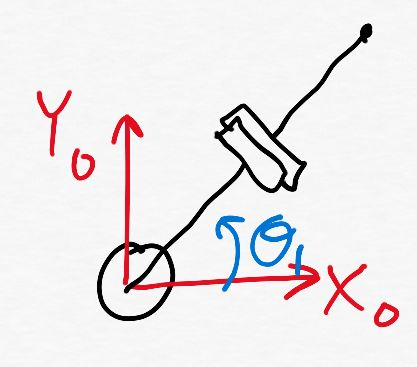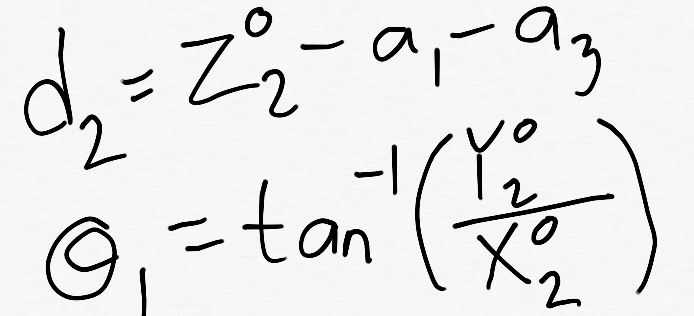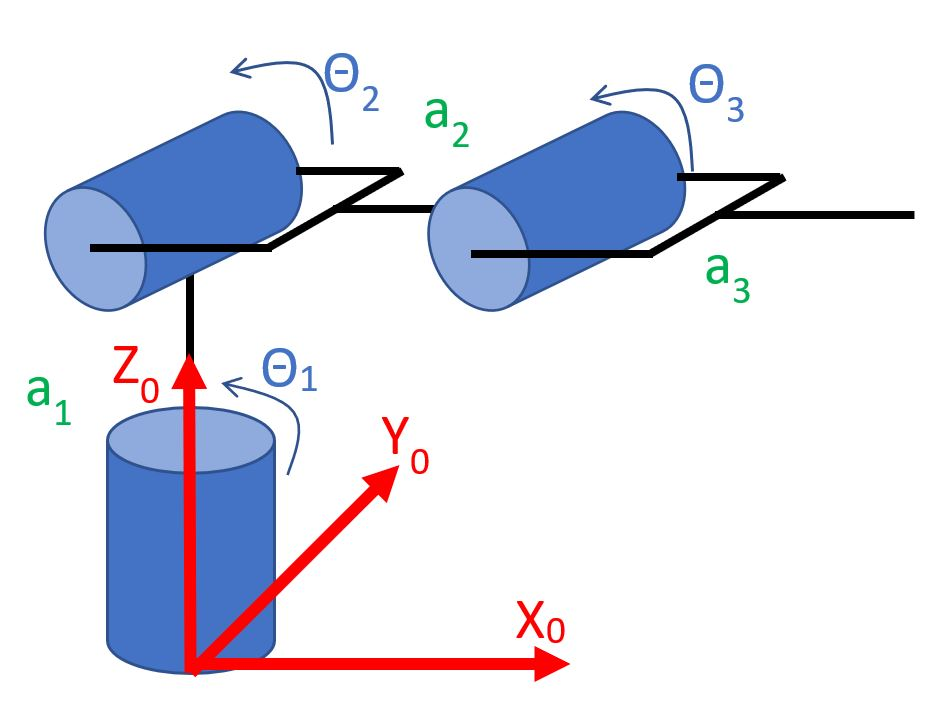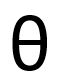Robotics 1
Inverse Kinematics for Position
QuizQuestion 2: When doing inverse kinematics, why don't we just find the homogeneous transformation matrix, and set the displacenment vector elements equal to the desired end-effector position?
Question 3:  Shown here is a kinematic diagram.  Which diagram shows the 'top view' of this manipulator?
Question 4: Which two triangle relationships are most useful to us when the triangle is a right triangle?
Question 6: In a set of inverse kinematics equations, which variables are the INPUT?
Question 7:  In a set of inverse kinematics equations, which variables are the OUTPUT?
Question 8: Shown here is a kinematic diagram.  Which inverse kinematics equations are correct?
Questions 9 and 10: Shown here is a kinematic diagram.  In this manipulator, our easiest approach to inverse kinematics is to first calculate the value of theta 2, then use that value to calculate d1.  Use the drop-down boxes to select two equations that will work for this approach.
Questions 11-15: Shown here is the kinematic diagram of an Articulated manipulator.  First, find the inverse kinematics equations.  Then, use your equations to find the combination of joint variable values that will put the end-effector in the specified positions.  To help you check your work, some of the answers have been given for you.  (Note: this is a pretty hard question.  If it takes a while for you to figure it out, don't get discouraged - push on, and do your best.)
Question 1: Which of these describes the 'inverse kinematics' problem?
We know the position and rotation of the base-frame, and we want to find the position and rotation of the end-effector frame.
We know the values of the joint variables, and we want to find the end-effector position and orientation.
We know the end-effector position and orientation, and we want to find the values of the joint variables.
This approach would require us to solve a nonlinear system of equations, which would require a numerical, rather than analytical, solution.
The pythagorean theorem and the law of sines
SOHCAHTOA and the law of sines
The law of sines and the law of cosines
The pythagorean theorem and SOHCAHTOA
The joint variables (theta and d)
The position of the end-effector (X, Y, Z)
The lengths of the links (a1, a2, a3, ...)
The type of manipulator (Cartesian, Articulated, ...)
We know the position and rotation of the end-effector frame, and we want to find the position and rotation of the base-frame.
This approach wouldn't give us the correct answer.  It is necessary to use both the top view and the side view of the manipulator.
There is no displacement vector in the homogeneous transformation matrix.
We would get the correct answer, but it wouldn't make sense for our manipulator.The pythagorean theorem and the law of sines
SOHCAHTOA and the law of sines
The law of sines and the law of cosines
Question 5: Which two triangle relationships are most useful to us when the triangle is NOT a right triangle?
The pythagorean theorem and SOHCAHTOA
The joint variables (theta and d)
The position of the end-effector (X, Y, Z)
The lengths of the links (a1, a2, a3, ...)
The type of manipulator (Cartesian, Articulated, ...)None of these is correct.
(9)
(10)a1=10 cm
a2=7 cm
a3=5 cm
Suppose that we would like the end-effector to be located at position 7.0 cm in the X0 direction, 4.0 cm in the Y0 direction, and 7.0 cm in the Z0 direction.  What values should we set for the three theta angles?  Round to the nearest degree.
= 15 degrees
=
degrees123
=
degrees
Suppose that we would like the end-effector to be located at position 3.0 cm in the X0 direction, 5.25 cm in the Y0 direction, and 8.5 cm in the Z0 direction.  What values should we set for the three theta angles?  Round to the nearest degree.1
=
degrees2
= -120 degrees3
=
degrees12
=
degrees3
Suppose that we would like the end-effector to be located at position 7.5 cm in the X0 direction, 6.3 cm in the Y0 direction, and 8.56 cm in the Z0 direction.  What values should we set for the three theta angles?  Round to the nearest degree.
= -70 degrees
= 40 degrees